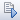»  Home »  Products »  Stata 13 »  Generalized SEM

## Generalized SEM

### Highlights

 Generalized linear responses Continuous—linear, log-gamma Binary—probit, logit, complementary log-log Count—Poisson, negative binomial Categorical—multinomial logit Ordered—ordered logit, ordered probit Censored continuous Multilevel data Nested: two levels, three levels, more levels Hierarchical Crossed Latent variables at different levels Random intercepts Random slopes (paths) Mixed models Meaning you can now fit CFA with binary, count, and ordinal measurements Multilevel CFA Multilevel mediation Item–response theory (IRT) Latent growth curves with repeated measurements of binary, count, and ordinal responses Selection models Endogenous treatment effects Any multilevel SEM with generalized linear responses

### Show me

In Stata 12, we introduced linear SEM.

In Stata 13, we introduce generalized SEM, by which we mean (1) SEM with generalized linear response variables and (2) SEM with multilevel mixed effects, whether linear or generalized linear.

Generalized linear response variables mean you can now fit logistic, probit, Poisson, multinomial logistic, ordered logit, ordered probit, and other models. It also means measurements can be continuous, binary, count, categorical, and ordered.

Multilevel mixed effects means you can place latent variables at different levels of the data. You can fit models with fixed or random intercepts and fixed or random slopes.

Say we have a test designed to assess mathematical performance. The data record a set of binary variables measuring whether individual answers were correct. The test was administered to students at various schools.

We postulate that performance on the questions is determined by unobserved (latent) mathematical aptitude and by school quality, representing unmeasured characteristics of the school:

In the diagram, the values of the latent variable SchQual are constant within school and vary across schools.

We can fit the model from the path diagram by pressing. Results will appear on the diagram.

Or, we can skip the diagram and type the equivalent command

. gsem ( MathApt -> q1 q2 q3 q4) (SchQual[school] -> q1 q2 q3 q4), logit

Either way, we get the same results:

Generalized structural equation model Number of obs = 2500 Log likelihood = -6506.1646 ( 1) [q1]SchQual[school] = 1 ( 2) [q2]MathApt = 1
 Coef. Std. Err. z P>|z| [95% Conf. Interval] q1 <- SchQual[school] 1 (constrained) MathApt 1.437913 .1824425 7.88 0.000 1.080333 1.795494 _cons .0459474 .1074647 0.43 0.669 -.1646795 .2565743 q2 <- SchQual[school] .1522361 .0823577 1.85 0.065 -.0091821 .3136543 MathApt 1 (constrained) _cons -.377969 .0518194 -7.29 0.000 -.4795332 -.2764047 q3 <- SchQual[school] .5194866 .0965557 5.38 0.000 .3302408 .7087324 MathApt .8650544 .1098663 7.87 0.000 .6497204 1.080388 _cons .026989 .0667393 0.40 0.686 -.1038175 .1577955 q4 <- SchQual[school] .6085149 .119537 5.09 0.000 .3742266 .8428032 MathApt 1.721957 .2466729 6.98 0.000 1.238487 2.205427 _cons -.3225736 .0845656 -3.81 0.000 -.4883191 -.1568281 var(SchQual[school]) .4167718 .1222884 .2344987 .7407238 var(MathApt) 1.004914 .1764607 .7122945 1.417744

Math aptitude has a larger variance and loadings than school quality. Thus math aptitude is more important than school, although school is still important.

### Show me more

See the entire 563-page Structural Equation Modeling Manual and especially see the introduction.We have added 18 new, worked examples demonstrating generalized SEM.

See New in Stata 17 to learn about what was added in Stata 17.